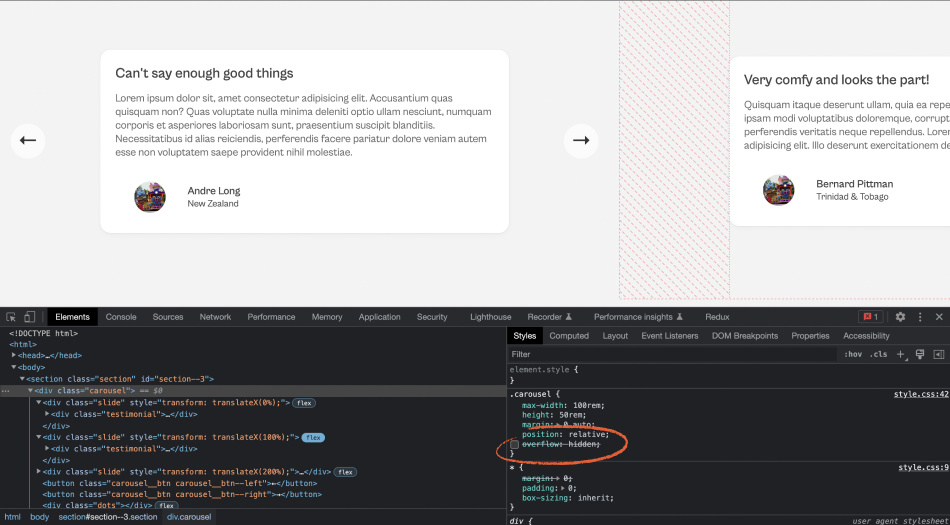# const - JS | lectureAdvanced DOM and Events

Building a Carousel Component Part 1

In this lecture and the next, we are going to build a carousel component. Below is a video of the final result of this lecture:

Before we start, head over to this link and download the starter files for this lecture. Right now, if you open the starter files in your browser, you will notice that all the slides are on top of each other. And so the first thing we should do is to establish an initial condition where they are all side by side. And for that, we need to translate each slide to a certain percentage. Let's get started!

script.js
``const slides = document.querySelectorAll('.slide');``

Let's now create the starting condition we mentioned earlier. i.e., putting all the slides side by side.

script.js
``````const slides = document.querySelectorAll('.slide');

// 0%, 100%, 200%
slides.forEach((slideEl, index) => {  slideEl.style.transform = `translateX(\${100 * index}%)`;});``````

If you want to see the slides side by side, you can just uncheck the `overflow: hidden` property directly in your browser.Now that we have all the slides side by side, let's now make our carousel work. And for that, let's start by selecting our buttons.

script.js
``````const carouselButtonLeft = document.querySelector('.carousel__btn--left');
const carouselButtonRight = document.querySelector('.carousel__btn--right');``````

Next, let's first try to make the slides move to the left when we click on the right button.

script.js
``````carouselButtonRight.addEventListener('click', function () {
// Go to the next slide
});``````

Remember that going to the next slide is simply changing the value of the `transform` property of each slide. i.e., we will change the percentage value we pass to the `translateX` function so that the slide that we want to move to is the one that has zero percent.

script.js
``````// We need to keep track of the current slide
let currentSlide = 0;

// Go to the next slide
currentSlide++;

// -100%, 0%, 100%
slides.forEach((slideEl, index) => {    slideEl.style.transform = `translateX(\${100 * (index - currentSlide)}%)`;  });});

/**
* An example in case you are confused
*
* let's say we are on the first slide (currentSlide = 0) and we click
* on the right button (currentSlide = 1)
*
* Our indexes are: 0, 1, 2
*
* When looping through the slides, we will have:
*
* 0 - 1 = -1 (were 0 is the index and 1 is the currentSlide)
* ==> -1 * 100 = -100%
*
* 1 - 1 = 0 (were 1 is the index and 1 is the currentSlide)
* ==> 0 * 100 = 0%
*
* 2 - 1 = 1 (were 2 is the index and 1 is the currentSlide)
* ==> 1 * 100 = 100%
*
* So the first slide will be translated to -100% and the second slide
* to 0% and the third slide to 100%
*
*/``````

With this in place, we can now click on the right button and see the slides move to the left. But you will notice that when we reach the last slide, and we keep on clicking on the right button, the slides will keep on moving to the left, and the translate values keep increasing. This happens because JavaScript does not know that we are working on a carousel and so it doesn't stop 😅. Hence, we need to tell JavaScript to stop when we reach the last slide.

To do that, the best way is to actually define the number of slides, and then make it stop when we reach the last slide.

script.js
``````const numberOfSlides = slides.length;

if (currentSlide >= numberOfSlides - 1) {    // Reset if we reach the last slide    currentSlide = 0;  } else {    // Go to the next slide    currentSlide++;  }
// -100%, 0%, 100%
slides.forEach((slideEl, index) => {
slideEl.style.transform = `translateX(\${100 * (index - currentSlide)}%)`;
});
});``````

Beautiful! Our slider is basically already working! At least in the right direction 😅. Before moving on, let's quickly refactor our code a bit. You will notice that these two pieces of code are basically the same.

script.js
``````// Code written inside the click event listener
slides.forEach((slideEl, index) => {
slideEl.style.transform = `translateX(\${100 * (index - currentSlide)}%)`;
});

// Code we wrote the align the slides side by side
slides.forEach((slideEl, index) => {
slideEl.style.transform = `translateX(\${100 * index}%)`;
});``````

Let's refactor this into its own function.

script.js
``````const updateSlidesPositions = function (position = 0) {
slides.forEach((slideEl, index) => {
slideEl.style.transform = `translateX(\${100 * (index - position)}%)`;
});
};``````

Now, we can call this function like this:

script.js
``````// On first load
// On click
if (currentSlide >= numberOfSlides - 1) {
// Reset if we reach the last slide
currentSlide = 0;
} else {
// Go to the next slide
currentSlide++;
}

We can even make it look more beautiful by completely exporting the event listener into its own named function.

script.js
``````const goToNextSlide = function () {
if (currentSlide >= numberOfSlides - 1) {
// Reset if we reach the last slide
currentSlide = 0;
} else {
// Go to the next slide
currentSlide++;
}

};

Next up, let's make the left button work.

script.js
``````const goToPreviousSlide = function () {
if (currentSlide <= 0) {
// Reset if we reach the first slide
currentSlide = numberOfSlides - 1;
} else {
// Go to the previous slide
currentSlide--;
}